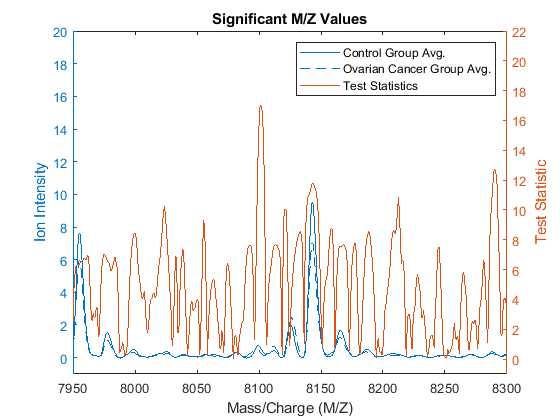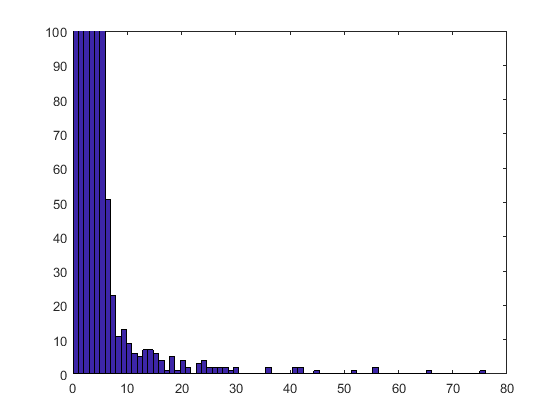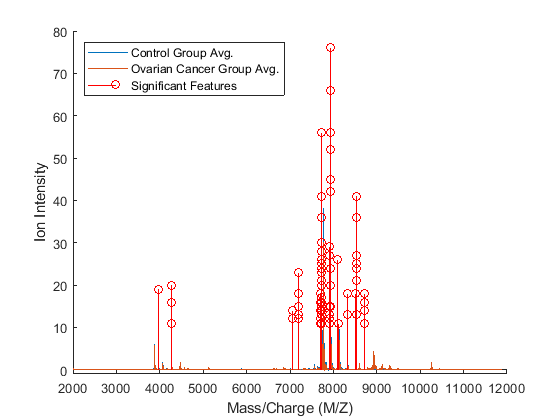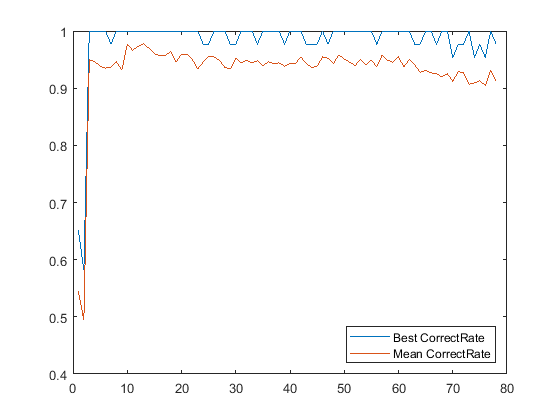# Identifying Significant Features and Classifying Protein Profiles

This example shows how to classify mass spectrometry data and use some statistical tools to look for potential disease markers and proteomic pattern diagnostics.

### Introduction

Serum proteomic pattern diagnostics can be used to differentiate samples from patients with and without disease. Profile patterns are generated using surface-enhanced laser desorption and ionization (SELDI) protein mass spectrometry. This technology has the potential to improve clinical diagnostics tests for cancer pathologies. The goal is to select a reduced set of measurements or "features" that can be used to distinguish between cancer and control patients. These features will be ion intensity levels at specific mass/charge values.

### Preprocess Data

The ovarian cancer data set in this example is from the FDA-NCI Clinical Proteomics Program Databank. The data set was generated using the WCX2 protein array. The data set includes 95 controls and 121 ovarian cancers. For a detailed description of this data set, see  and .

This example assumes that you already have the preprocessed data `OvarianCancerQAQCdataset.mat`. However, if you do not have the data file, you can recreate by following the steps in the example Batch Processing of Spectra Using Sequential and Parallel Computing.

Alternatively, you can run the provided script `msseqprocessing.m`.

The preprocessing steps from the script and example listed above are intended to illustrate a representative set of possible pre-processing procedures. Using different steps or parameters may lead to different and possibly improved results of this example.

Once you have the preprocessed data, you can load it into MATLAB.

```load OvarianCancerQAQCdataset whos ```
``` Name Size Bytes Class Attributes MZ 15000x1 120000 double Y 15000x216 25920000 double grp 216x1 25056 cell ```

There are three variables: MZ, Y, grp. MZ is the mass/charge vector, Y is the intensity values for all 216 patients (control and cancer), and grp holds the index information as to which of these samples represent cancer patients and which ones represent normal patients.

Initialize some variables that will be used through out the example.

```N = numel(grp); % Number of samples Cidx = strcmp('Cancer',grp); % Logical index vector for Cancer samples Nidx = strcmp('Normal',grp); % Logical index vector for Normal samples Cvec = find(Cidx); % Index vector for Cancer samples Nvec = find(Nidx); % Index vector for Normal samples xAxisLabel = 'Mass/Charge (M/Z)'; % x label for plots yAxisLabel = 'Ion Intensity'; % y label for plots ```

### Visualizing Some of the Samples

You can plot some data sets into a figure window to visually compare profiles from the two groups; in this example five spectrograms from cancer patients (blue) and five from control patients (green) are displayed.

```figure; hold on; hC = plot(MZ,Y(:,Cvec(1:5)),'b'); hN = plot(MZ,Y(:,Nvec(1:5)),'g'); xlabel(xAxisLabel); ylabel(yAxisLabel); axis([2000 12000 -5 60]) legend([hN(1),hC(1)],{'Control','Ovarian Cancer'}) title('Multiple Sample Spectrograms') ```Zooming in on the region from 8500 to 8700 M/Z shows some peaks that might be useful for classifying the data.

```axis([8450,8700,-1,7]) ```Another way to visualize the whole data set is to look at the group average signal for the control and cancer samples. You can plot the group average and the envelopes of each group.

```mean_N = mean(Y(:,Nidx),2); % group average for control samples max_N = max(Y(:,Nidx),[],2); % top envelopes of the control samples min_N = min(Y(:,Nidx),[],2); % bottom envelopes of the control samples mean_C = mean(Y(:,Cidx),2); % group average for cancer samples max_C = max(Y(:,Cidx),[],2); % top envelopes of the control samples min_C = min(Y(:,Cidx),[],2); % bottom envelopes of the control samples figure; hold on; hC = plot(MZ,mean_C,'b'); hN = plot(MZ,mean_N,'g'); gC = plot(MZ,[max_C min_C],'b--'); gN = plot(MZ,[max_N min_N],'g--'); xlabel(xAxisLabel); ylabel(yAxisLabel); axis([8450,8700,-1,7]) legend([hN,hC,gN(1),gC(1)],{'Control Group Avg.','Ovarian Cancer Group Avg',... 'Control Envelope','Ovarian Cancer Envelope'},... 'Location', 'NorthWest') title('Group Average and Group Envelopes') ```Observe that apparently there is no single feature that can discriminate both groups perfectly.

### Ranking Key Features

A simple approach for finding significant features is to assume that each M/Z value is independent and compute a two-way t-test. `rankfeatures` returns an index to the most significant M/Z values, for instance 100 indices ranked by the absolute value of the test statistic. This feature selection method is also known as a filtering method, where the learning algorithm is not involved on how the features are selected.

```[feat,stat] = rankfeatures(Y,grp,'CRITERION','ttest','NUMBER',100); ```

The first output of `rankfeatures` can be used to extract the M/Z values of the significant features.

```sig_Masses = MZ(feat); sig_Masses(1:7)' %display the first seven ```
```ans = 1.0e+03 * 8.1009 8.1016 8.1024 8.1001 8.1032 7.7366 7.7359 ```

The second output of `rankfeatures` is a vector with the absolute value of the test statistic. You can plot it over the spectra using `yyaxis`.

```figure; yyaxis left plot(MZ, [mean_N mean_C]); ylim([-1,20]) xlim([7950,8300]) title('Significant M/Z Values') xlabel(xAxisLabel); ylabel(yAxisLabel); yyaxis right plot(MZ,stat); ylim([-1,22]) ylabel('Test Statistic'); legend({'Control Group Avg.','Ovarian Cancer Group Avg.', 'Test Statistics'}) ```Notice that there are significant regions at high M/Z values but low intensity (~8100 Da.). Other approaches to measure class separability are available in `rankfeatures`, such as entropy based, Bhattacharyya, or the area under the empirical receiver operating characteristic (ROC) curve.

### Blind Classification Using Linear Discriminant Analysis (LDA)

Now that you have identified some significant features, you can use this information to classify the cancer and normal samples. Due to the small number of samples, you can run a cross-validation using the 20% holdout to have a better estimation of the classifier performance. `cvpartition` allows you to set the training and test indices for different types of system evaluation methods, such as hold-out, K-fold and Leave-M-Out.

```per_eval = 0.20; % training size for cross-validation rng('default'); % initialize random generator to the same state % used to generate the published example cv = cvpartition(grp,'holdout',per_eval) ```
```cv = Hold-out cross validation partition NumObservations: 216 NumTestSets: 1 TrainSize: 173 TestSize: 43 IsCustom: 0 ```

Observe that features are selected only from the training subset and the validation is performed with the test subset. `classperf` allows you to keep track of multiple validations.

```cp_lda1 = classperf(grp); % initializes the CP object for k=1:10 % run cross-validation 10 times cv = repartition(cv); feat = rankfeatures(Y(:,training(cv)),grp(training(cv)),'NUMBER',100); c = classify(Y(feat,test(cv))',Y(feat,training(cv))',grp(training(cv))); classperf(cp_lda1,c,test(cv)); % updates the CP object with current validation end ```

After the loop you can assess the performance of the overall blind classification using any of the properties in the CP object, such as the error rate, sensitivity, specificity, and others.

```cp_lda1 ```
```cp_lda1 = classperformance with properties: ClassLabels: {2×1 cell} GroundTruth: [216×1 double] NumberOfObservations: 216 ValidationCounter: 10 SampleDistribution: [216×1 double] ErrorDistribution: [216×1 double] SampleDistributionByClass: [2×1 double] ErrorDistributionByClass: [2×1 double] CountingMatrix: [3×2 double] CorrectRate: 0.8488 ErrorRate: 0.1512 LastCorrectRate: 0.8837 LastErrorRate: 0.1163 InconclusiveRate: 0 ClassifiedRate: 1 Sensitivity: 0.8208 Specificity: 0.8842 PositivePredictiveValue: 0.8995 NegativePredictiveValue: 0.7962 PositiveLikelihood: 7.0890 NegativeLikelihood: 0.2026 Prevalence: 0.5581 DiagnosticTable: [2×2 double] Label: '' Description: '' ControlClasses: 2 TargetClasses: 1 ```

This naive approach for feature selection can be improved by eliminating some features based on the regional information. For example, 'NWEIGHT' in `rankfeatures` outweighs the test statistic of neighboring M/Z features such that other significant M/Z values can be incorporated into the subset of selected features

```cp_lda2 = classperf(grp); % initializes the CP object for k=1:10 % run cross-validation 10 times cv = repartition(cv); feat = rankfeatures(Y(:,training(cv)),grp(training(cv)),'NUMBER',100,'NWEIGHT',5); c = classify(Y(feat,test(cv))',Y(feat,training(cv))',grp(training(cv))); classperf(cp_lda2,c,test(cv)); % updates the CP object with current validation end cp_lda2.CorrectRate % average correct classification rate ```
```ans = 0.9023 ```

### PCA/LDA Reduction of the Data Dimensionality

Lilien et al. presented in  an algorithm to reduce the data dimensionality that uses principal component analysis (PCA), then LDA is used to classify the groups. In this example 2000 of the most significant features in the M/Z space are mapped to the 150 principal components

```cp_pcalda = classperf(grp); % initializes the CP object for k=1:10 % run cross-validation 10 times cv = repartition(cv); % select the 2000 most significant features. feat = rankfeatures(Y(:,training(cv)),grp(training(cv)),'NUMBER',2000); % PCA to reduce dimensionality P = pca(Y(feat,training(cv))'); % Project into PCA space x = Y(feat,:)' * P(:,1:150); % Use LDA c = classify(x(test(cv),:),x(training(cv),:),grp(training(cv))); classperf(cp_pcalda,c,test(cv)); end cp_pcalda.CorrectRate % average correct classification rate ```
```ans = 0.9814 ```

### Randomized Search for Subset Feature Selection

Feature selection can also be reinforced by classification, this approach is usually referred to as a wrapper selection method. Randomized search for feature selection generates random subsets of features and assesses their quality independently with the learning algorithm. Later, it selects a pool of the most frequent good features. Li et al. in  apply this concept to the analysis of protein expression patterns. The `randfeatures` function allows you to search a subset of features using LDA or a k-nearest neighbor classifier over randomized subsets of features.

Note: the following example is computationally intensive, so it has been disabled from the example. Also, for better results you should increase the pool size and the stringency of the classifier from the default values in `randfeatures`. Type `help randfeatures` for more information.

```if 0 % <== change to 1 to enable. This may use extensive time to complete. cv = repartition(cv); [feat,fCount] = randfeatures(Y(:,training(cv)),grp(training(cv)),... 'CLASSIFIER','da','PerformanceThreshold',0.90); else load randFeatCancerDetect end ```

### Assess the Quality of the Selected Features with the Evaluation Set

The first output from `randfeatures` is an ordered list of indices of MZ values. The first item occurs most frequently in the subsets where good classification was achieved. The second output is the actual counts of the number of times each value was selected. You can use `hist` to look at this distribution.

```figure; hist(fCount,max(fCount)+1); ```You will see that most values appear at most once in a selected subset. Zooming in gives a better idea of the details for the more frequently selected values.

```axis([0 80 0 100]) ```Only a few values were selected more than 10 times. You can visualize these by using a stem plot to show the most frequently selected features.

```figure; hold on; sigFeats = fCount; sigFeats(sigFeats<=10) = 0; plot(MZ,[mean_N mean_C]); stem(MZ(sigFeats>0),sigFeats(sigFeats>0),'r'); axis([2000,12000,-1,80]) legend({'Control Group Avg.','Ovarian Cancer Group Avg.','Significant Features'}, ... 'Location', 'NorthWest') xlabel(xAxisLabel); ylabel(yAxisLabel); ```These features appear to clump together in several groups. You can investigate further how many of the features are significant by running the following experiment. The most frequently selected feature is used to classify the data, then the two most frequently selected features are used and so on until all the features that were selected more than 10 times are used. You can then see if adding more features improves the classifier.

```nSig = sum(fCount>10); cp_rndfeat = zeros(20,nSig); for i = 1:nSig for j = 1:20 cv = repartition(cv); P = pca(Y(feat(1:i),training(cv))'); x = Y(feat(1:i),:)' * P; c = classify(x(test(cv),:),x(training(cv),:),grp(training(cv))); cp = classperf(grp,c,test(cv)); cp_rndfeat(j,i) = cp.CorrectRate; % average correct classification rate end end figure plot(1:nSig, [max(cp_rndfeat);mean(cp_rndfeat)]); legend({'Best CorrectRate','Mean CorrectRate'},'Location', 'SouthEast') ```From this graph you can see that for as few as three features it is sometimes possible to get perfect classification. You will also notice that the maximum of the mean correct rate occurs for a small number of features and then gradually decreases.

```[bestAverageCR, bestNumFeatures] = max(mean(cp_rndfeat)); ```

You can now visualize the features that give the best average classification. You can see that these actually correspond to only three peaks in the data.

```figure; hold on; sigFeats = fCount; sigFeats(sigFeats<=10) = 0; ax_handle = plot(MZ,[mean_N mean_C]); stem(MZ(feat(1:bestNumFeatures)),sigFeats(feat(1:bestNumFeatures)),'r'); axis([7650,8850,-1,80]) legend({'Control Group Avg.','Ovarian Cancer Group Avg.','Significant Features'}) xlabel(xAxisLabel); ylabel(yAxisLabel); ```### Alternative Statistical Learning Algorithms

There are many classification tools in MATLAB® that you can also use to analyze proteomic data. Among them are support vector machines (`fitcsvm`), k-nearest neighbors (`fitcknn`), neural networks (Deep Learning Toolbox™), and classification trees (`fitctree`). For feature selection, you can also use sequential subset feature selection (`sequentialfs`) or optimize the randomized search methods by using a genetic algorithm (Global Optimization Toolbox). For example, see Genetic Algorithm Search for Features in Mass Spectrometry Data.

 Conrads, T P, V A Fusaro, S Ross, D Johann, V Rajapakse, B A Hitt, S M Steinberg, et al. “High-Resolution Serum Proteomic Features for Ovarian Cancer Detection.” Endocrine-Related Cancer, June 2004, 163–78.

 Lilien, Ryan H., Hany Farid, and Bruce R. Donald. “Probabilistic Disease Classification of Expression-Dependent Proteomic Data from Mass Spectrometry of Human Serum.” Journal of Computational Biology 10, no. 6 (December 2003): 925–46.

 Li, L., D. M. Umbach, P. Terry, and J. A. Taylor. “Application of the GA/KNN Method to SELDI Proteomics Data.” Bioinformatics 20, no. 10 (July 1, 2004): 1638–40.

 Petricoin, Emanuel F, Ali M Ardekani, Ben A Hitt, Peter J Levine, Vincent A Fusaro, Seth M Steinberg, Gordon B Mills, et al. “Use of Proteomic Patterns in Serum to Identify Ovarian Cancer.” The Lancet 359, no. 9306 (February 2002): 572–77.